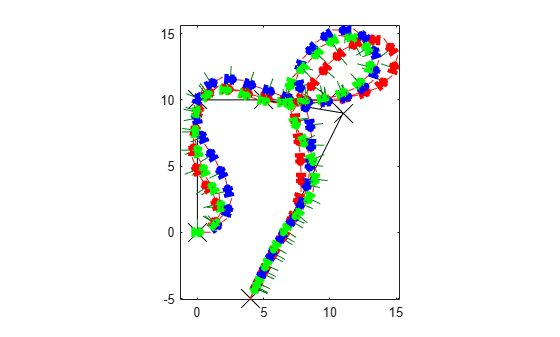## Simulate Different Kinematic Models for Mobile Robots

This example shows how to model different robot kinematics models in an environment and compare them.

### Define Mobile Robots with Kinematic Constraints

There are a number of ways to model the kinematics of mobile robots. All dictate how the wheel velocities are related to the robot state: `[x y theta]`, as xy-coordinates and a robot heading, `theta`, in radians.

#### Unicycle Kinematic Model

The simplest way to represent mobile robot vehicle kinematics is with a unicycle model, which has a wheel speed set by a rotation about a central axle, and can pivot about its z-axis. Both the differential-drive and bicycle kinematic models reduce down to unicycle kinematics when inputs are provided as vehicle speed and vehicle heading rate and other constraints are not considered.

`unicycle = unicycleKinematics("VehicleInputs","VehicleSpeedHeadingRate");`

#### Differential-Drive Kinematic Model

The differential drive model uses a rear driving axle to control both vehicle speed and head rate. The wheels on the driving axle can spin in both directions. Since most mobile robots have some interface to the low-level wheel commands, this model will again use vehicle speed and heading rate as input to simplify the vehicle control.

`diffDrive = differentialDriveKinematics("VehicleInputs","VehicleSpeedHeadingRate");`

To differentiate the behavior from the unicycle model, add a wheel speed velocity constraint to the differential-drive kinematic model

`diffDrive.WheelSpeedRange = [-10 10]*2*pi;`

#### Bicycle Kinematic Model

The bicycle model treats the robot as a car-like model with two axles: a rear driving axle, and a front axle that turns about the z-axis. The bicycle model works under the assumption that wheels on each axle can be modeled as a single, centered wheel, and that the front wheel heading can be directly set, like a bicycle.

`bicycle = bicycleKinematics("VehicleInputs","VehicleSpeedHeadingRate","MaxSteeringAngle",pi/8);`

#### Other Models

The Ackermann kinematic model is a modified car-like model that assumes Ackermann steering. In most car-like vehicles, the front wheels do not turn about the same axis, but instead turn on slightly different axes to ensure that they ride on concentric circles about the center of the vehicle's turn. This difference in turning angle is called Ackermann steering, and is typically enforced by a mechanism in actual vehicles. From a vehicle and wheel kinematics standpoint, it can be enforced by treating the steering angle as a rate input.

`carLike = ackermannKinematics;`

### Set up Simulation Parameters

These mobile robots will follow a set of waypoints that is designed to show some differences caused by differing kinematics.

```waypoints = [0 0; 0 10; 10 10; 5 10; 11 9; 4 -5]; % Define the total time and the sample rate sampleTime = 0.05; % Sample time [s] tVec = 0:sampleTime:20; % Time array initPose = [waypoints(1,:)'; 0]; % Initial pose (x y theta)```

### Create a Vehicle Controller

The vehicles follow a set of waypoints using a Pure Pursuit controller. Given a set of waypoints, the robot current state, and some other parameters, the controller outputs vehicle speed and heading rate.

```% Define a controller. Each robot requires its own controller controller1 = controllerPurePursuit("Waypoints",waypoints,"DesiredLinearVelocity",3,"MaxAngularVelocity",3*pi); controller2 = controllerPurePursuit("Waypoints",waypoints,"DesiredLinearVelocity",3,"MaxAngularVelocity",3*pi); controller3 = controllerPurePursuit("Waypoints",waypoints,"DesiredLinearVelocity",3,"MaxAngularVelocity",3*pi);```

### Simulate the Models Using an ODE Solver

The models are simulated using the `derivative` function to update the state. This example uses an ordinary differential equation (ODE) solver to generate a solution. Another way would be to update the state using a loop, as shown in Path Following for a Differential Drive Robot.

Since the ODE solver requires all outputs to be provided as a single output, the pure pursuit controller must be wrapped in a function that outputs the linear velocity and heading angular velocity as a single output. An example helper, `exampleHelperMobileRobotController`, is used for that purpose. The example helper also ensures that the robot stops when it is within a specified radius of the goal.

```goalPoints = waypoints(end,:)'; goalRadius = 1;```

`ode45` is called once for each type of model. The derivative function computes the state outputs with initial state set by `initPose`. Each derivative accepts the corresponding kinematic model object, the current robot pose, and the output of the controller at that pose.

```% Compute trajectories for each kinematic model under motion control [tUnicycle,unicyclePose] = ode45(@(t,y)derivative(unicycle,y,exampleHelperMobileRobotController(controller1,y,goalPoints,goalRadius)),tVec,initPose); [tBicycle,bicyclePose] = ode45(@(t,y)derivative(bicycle,y,exampleHelperMobileRobotController(controller2,y,goalPoints,goalRadius)),tVec,initPose); [tDiffDrive,diffDrivePose] = ode45(@(t,y)derivative(diffDrive,y,exampleHelperMobileRobotController(controller3,y,goalPoints,goalRadius)),tVec,initPose);```

### Plot Results

The results of the ODE solver can be easily viewed on a single plot using `plotTransforms` to visualize the results of all trajectories at once.

The pose outputs must first be converted to indexed matrices of translations and quaternions.

```unicycleTranslations = [unicyclePose(:,1:2) zeros(length(unicyclePose),1)]; unicycleRot = axang2quat([repmat([0 0 1],length(unicyclePose),1) unicyclePose(:,3)]); bicycleTranslations = [bicyclePose(:,1:2) zeros(length(bicyclePose),1)]; bicycleRot = axang2quat([repmat([0 0 1],length(bicyclePose),1) bicyclePose(:,3)]); diffDriveTranslations = [diffDrivePose(:,1:2) zeros(length(diffDrivePose),1)]; diffDriveRot = axang2quat([repmat([0 0 1],length(diffDrivePose),1) diffDrivePose(:,3)]);```

Next, the set of all transforms can be plotted and viewed from the top. The paths of the unicycle, bicycle, and differential-drive robots are red, blue, and green, respectively. To simplify the plot, only show every tenth output.

```figure plot(waypoints(:,1),waypoints(:,2),"kx-","MarkerSize",20); hold all plotTransforms(unicycleTranslations(1:10:end,:),unicycleRot(1:10:end,:),'MeshFilePath','groundvehicle.stl',"MeshColor","r"); plotTransforms(bicycleTranslations(1:10:end,:),bicycleRot(1:10:end,:),'MeshFilePath','groundvehicle.stl',"MeshColor","b"); plotTransforms(diffDriveTranslations(1:10:end,:),diffDriveRot(1:10:end,:),'MeshFilePath','groundvehicle.stl',"MeshColor","g"); view(0,90)```##### Support평가판 신청